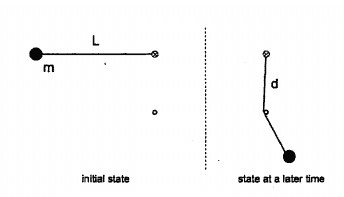# Angular momentum conservation in collision with a nail

## Homework Statement

A ball of mass ##m## is attached to a massless string of length ##L##. The ball is released from rest as shown in the figure and as it reaches the bottom of the circle, the string wraps around a nail which is a distance ##d## below the center of the circle. What is the velocity of the ball after the collision?## Homework Equations

The velocity before the collision is ##v=\sqrt{2gL}## from energy conservation.
From angular momentum conservation around the nail ##(L-d)mv=(L-d)mv_{f}##.

## The Attempt at a Solution

So the velocity after the collision is the same as before the collision. Is this right?

#### Attachments

ZapperZ
Staff Emeritus
From angular momentum conservation around the nail ##(L-d)mv=(L-d)mv_{f}##.

Think again. This scenario is the same as if the length of the pendulum is shortened instantaneously. Where is the pivot point of the pendulum before it hits the nail, and where is the new pivot point for the pendulum after it hits the nail? Your equation here is indicating that the pivot point remains the same before and after, which is wrong.

Zz.

Think again. This scenario is the same as if the length of the pendulum is shortened instantaneously. Where is the pivot point of the pendulum before it hits the nail, and where is the new pivot point for the pendulum after it hits the nail? Your equation here is indicating that the pivot point remains the same before and after, which is wrong.

Zz.
You are saying that it should be ##Lmv=(L-d)mv_{f}##? But then I would not be computing the angular momentum about the same pivot and how can I talk about angular momentum conservation then?

ZapperZ
Staff Emeritus
You are saying that it should be ##Lmv=(L-d)mv_{f}##? But then I would not be computing the angular momentum about the same pivot and how can I talk about angular momentum conservation then?

Where is the requirement that the pivot point must be at the same location in the conservation of angular momentum in this case?

Zz.

Where is the requirement that the pivot point must be at the same location in the conservation of angular momentum in this case?

Zz.
There is none but I was under the (probably false) impression that when we talk about conservation of angular momentum we compute the momentum before and after relative to the same point (like in linear momentum conservation collision problems). Perhaps energy conservation is a better mental model for me. Also I thought that I could invoke conservation of horizontal linear momentum at the bottom of the circle in which case the velocities would be the same before and after.

Last edited:
ZapperZ
Staff Emeritus
There is none but I was under the (probably false) impression that when we talk about conservation of angular momentum we compute the momentum before and after relative to the same point (like in linear momentum conservation collision problems). Perhaps energy conservation is a better mental model for me.

But as I've stated earlier, this is no different than shortening the length of the pendulum instantaneously when it hits the nail! So what is "oscillating" has one length before, and one length after. That is all that matters.

Your equation makes no sense, because the "before" has the same length of pendulum as the "after". You are making the pendulum having identical lengths before and after. This is not the scenario of your problem, i.e. your equation doesn't match what is going on.

Zz.

There is none but I was under the (probably false) impression that when we talk about conservation of angular momentum we compute the momentum before and after relative to the same point (like in linear momentum conservation collision problems). Perhaps energy conservation is a better mental model for me.
But as I've stated earlier, this is no different than shortening the length of the pendulum instantaneously when it hits the nail! So what is "oscillating" has one length before, and one length after. That is all that matters.

Your equation makes no sense, because the "before" has the same length of pendulum as the "after". You are making the pendulum having identical lengths before and after. This is not the scenario of your problem, i.e. your equation doesn't match what is going on.

Zz.
I have conservation of horizontal momentum at the bottom of the circle? In which case the velocity after would be the same as before?

ZapperZ
Staff Emeritus
I have conservation of horizontal momentum at the bottom of the circle? In which case the velocity after would be the same as before?

Why, especially when the length of the pendulum is different after collision with the nail?

Zz.

jbriggs444
Homework Helper
There is none but I was under the (probably false) impression that when we talk about conservation of angular momentum we compute the momentum before and after relative to the same point (like in linear momentum conservation collision problems). Perhaps energy conservation is a better mental model for me. Also I thought that I could invoke conservation of horizontal linear momentum at the bottom of the circle in which case the velocities would be the same before and after.
Possibly you are overthinking this.

The elapsed time between "just before the collision" to "just after the collision" can be as small as we please. By inspection there are no infinite forces and no infinite distances. So there can be no significant changes to momentum, energy or angular momentum about any axis.

haruspex